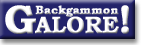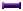Match EquitiesTurner formula

 From: Stephen Turner Address: sret1@statslab.cam.ac.uk Date: 22 June 1994 Subject: Turner formula, or Equities Easily Forum: rec.games.backgammon Google: 2u8tib\$n35@lyra.csx.cam.ac.uk

```Have problems remembering or working out those match equities? Then
use the new Turner formula!

The match equity is defined as the probability that a given player
will win the match, given the match score, and assuming perfect play
by both players. Kit Woolsey in his book "How to Play Tournament
Backgammon" has a table of these equities, for all scores where
neither player needs more than 15 points to win. They are useful in
making doubling decisions.

Rick Janowski invented a formula, known (unsurprisingly!) as the
Janowski formula, for working out these equities pretty accurately
without having to memorise them all. It is defined as follows.
Let L = number of points the leader still needs;
T = number of points the trailer needs;
D = the difference, i.e. (T - L).
Then the leader's equity in % is approximately

85 x D
50 +  --------
T + 6

For example, the equity at 3-away, 8-away is
50 + (5 x 85) / (8 + 6)
=  50 + 425 / 14
=  80% to the nearest %, which agrees with Kit's table.

The only problem with Janowski's formula is that many people find it
hard to work out 425/14, even to the nearest 1, in their heads. Hence
I have invented the following formula which is much easier to
calculate. The Turner formula is

50 + (24/T + 3) x D

To take the above example again, we divide 24 by T ( = 8) to get 3,
add 3 to get 6, multiply by D ( = 5) to get 30 and add 50 to get 80,
the same result as before.

You will notice that the above example comes out in whole numbers.
This is not just because it was a well chosen example. Because 24 is
such a nice number, my formula comes out in whole numbers when the
trailer is 3-away, 4-away, 6-away, 8-away or 12-away. The other
numbers up to 12 are not too bad either: 24/5 = 4.8; 24/7 = nearly 3 1/2;
24/9 = 2 2/3; 24/10 = 2.4 and 24/11 = 2 2/11. For example, at
3-away, 7-away, the Turner formula has (24/7 + 3) = nearly 6.5,
multiply by 4 to get nearly 26, so the equity is nearly 76. Janowski's
formula gives 50 + 340/13 = about 76.2, Kit's table gives 76.

Limitations:
1) Neither formula works when L = 1, i.e. in the Crawford game. You'll
just have to learn those. (You don't need to know about post-Crawford;
there's no doubling decisions to be made that affect that).
2) Both formulae underestimate when L = 2, and 5 <= T <= 9. You might
like to add 2% to these equities with either formula if you're worried.
3) When T >= 13, neither formula is great but mine is bad. Also the
arithmetic in mine isn't any easier by then. If you're playing long
matches, you might find Janowski better at the beginning of the match
(or when one of you is still at the beginning!)
4) My formula does worse than Janowski's when T = 11 and L = 2, 3; also
when T = 12 and L = 2, 3, 4. All these equities are in the 90-95%
range anyway.

Apart from these few cases, however, my formula is accurate to within
1% for all equities where T <= 12, and, I claim, is much easier to
calculate than Janowski's in all those cases.
--
Stephen Turner
Stochastic Networks Group, Statistical Laboratory,
University of Cambridge, CB2 1SB,  England
e-mail: S.R.E.Turner@statslab.cam.ac.uk

PS  Many thanks to Kit Woolsey for commenting on the above post. He
described to me another system called Neil's Numbers, invented by Neil
Kazaross, which is again basically the same, but you learn a short
table of multipliers instead of using my (24/T + 3) or Janowski's
85 / (T + 6). The table is

T    3  4  5  6  7  8  9  10  11  12  13  14  15
multiplier   10  9  8  7     6          5               4

Interpolate where no number is given. I think my method is easiest up
to T=12, but use one of the other two if you prefer!
S.T.
```

### Match Equities

Constructing a match equity table  (Walter Trice, Apr 2000)
Does it matter which match equity table you use?  (Klaus Evers+, Nov 2005)Does it matter which match equity table you use?  (Achim Mueller+, Dec 2003)
Does it matter which match equity table you use?  (Chuck Bower+, Sept 2001)ME Table: Big Brother  (Peter Fankhauser, July 1996)
ME Table: Dunstan  (Ian Dunstan+, Aug 2004)ME Table: Escoffery  (David Escoffery, Nov 1991)
ME Table: Friedman  (Elliott C Winslow, Oct 1991)
ME Table: Kazaross  (Neil Kazaross, Dec 2003)ME Table: Kazaross-XG2  (neilkaz, Aug 2011)
ME Table: Rockwell-Kazaross  (Chuck Bower+, June 2010)
ME Table: Snowie  (Chase, Apr 2002)
ME Table: Snowie  (Harald Retter, Aug 1998)
ME Table: Woolsey  (Raccoon, Apr 2006)
ME Table: Woolsey  (Kit Woolsey, May 1994)
ME Table: Woolsey  (William R. Tallmadge, Jan 1994)
ME Table: Zadeh  (Jørn Thyssen, Mar 2004)ME Table: Zorba  (Robert-Jan Veldhuizen+, Dec 2003)
ME at 1-away/2-away (crawford)  (Fabrice Liardet+, Nov 2007)ME at 1-away/2-away (crawford)  (Ian Shaw+, Apr 2003)Match equities--an alternate view  (Durf Freund, Oct 1994)
Neil's new numbers  (neilkaz, Aug 2011)
Neil's numbers  (Kit Woolsey+, Oct 1994)
On calculating match equity tables  (Neil Kazaross, July 2004)Turner formula  (Gregg Cattanach, Feb 2003)
Turner formula  (Stephen Turner, June 1994)
Using a match equity table  (Michael J. Zehr, June 1992)
Value of free drop  (Neil Kazaross, Oct 2002)Which match equity table is best?  (Martin Krainer+, Oct 2003)
Which match equity table is best?  (Ian Shaw+, Dec 2001)
Why use a match equity table?  (Kit Woolsey, Feb 1999)
Worth memorizing?  (Alef Rosenbaum+, Feb 2003)From GammOnLineLong messageRecommended readingRecent addition

 Book Suggestions Books Cheating Chouettes Computer Dice Cube Handling Cube Handling in Races Equipment Etiquette Extreme Gammon Fun and frustration GNU Backgammon History Jellyfish Learning Luck versus Skill Magazines & E-zines Match Archives Match Equities Match Play Match Play at 2-away/2-away Miscellaneous Opening Rolls Pip Counting Play Sites Probability and Statistics Programming Propositions Puzzles Ratings Rollouts Rules Rulings Snowie Software Source Code Strategy--Backgames Strategy--Bearing Off Strategy--Checker play Terminology Theory Tournaments Uncategorized Variations